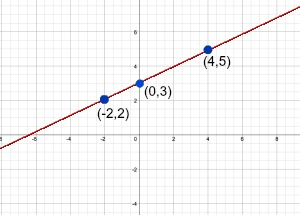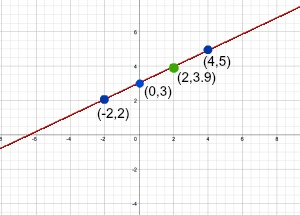# Collinear in Geometry: Definition & Example

An error occurred trying to load this video.

Try refreshing the page, or contact customer support.

Coming up next: Ratios & Rates: Definitions & Examples

### You're on a roll. Keep up the good work!

Replay
Your next lesson will play in 10 seconds
• 0:00 Collinear Points
• 0:57 Determining Collinear Points
• 2:48 Using the Slope of a Line
• 5:41 Lesson Summary

Want to watch this again later?

Timeline
Autoplay
Autoplay
Speed

#### Recommended Lessons and Courses for You

Lesson Transcript
Instructor: Laura Pennington

Laura has taught collegiate mathematics and holds a master's degree in pure mathematics.

In this lesson, we explore the definition of collinear points and how to recognize them in our environment. We learn how to determine if a given set of points are collinear by exploring graphs and slopes.

## Collinear Points

It is incredibly common to look up and see a bunch of birds lined up, perched on a group of power lines. Have you ever wondered how they do this without getting shocked? The fact is that birds don't get shocked while sitting on the power lines, because they are not good conductors of electricity compared to the wire. For this reason, the electricity passes right by them and continues along the wire.

Another interesting fact about seeing birds on these power lines is that the set of birds on each individual power line can be said to be collinear. In mathematics, a set of points that all lie on the same line are collinear. When you see birds all lined up along a power line, you see that they all lie on the same line. Another example of collinear points would be musical notes on sheet music. We see that musical notes of the same key lie on the same barline, making them collinear.

## Determining Collinear Points

Mathematically speaking, it's easy to see that any two points are collinear. This is because you can draw a line through any two points, so they must lie on the same line. But how do we tell if a set of points are collinear if there are more than two of them? For instance, if I gave you the points (0,3), (4,5), and (-2, 2), how can we determine if they all lie on the same line? One way to do this would be to graph them and see if they all lie on the same line.In this example, it appears that the three points are collinear, and in fact, they are. However, the downside to this is that you can sometimes get a point that is very close to a line and looks like it lies on it, but it actually doesn't. For instance, in our example, the three points all lie on the line y = ½x + 3. What if I added the point (2, 3.9) to the set, and I wanted to know if the points (0,3), (4,5), (-2,2), and (2, 3.9) were all collinear. If we plotted this extra point on the graph, it would appear that it fell on this line.However, it actually doesn't, because it doesn't satisfy the equation of the line. If we plug in 2 for x, then our y ends up being (1/2) * 2 + 3 = 4, not 3.9. Thus, in reality, the point doesn't lie on the same line, so the set of those four points would not be collinear. So, how can we tell for sure? The answer lies in the slope of a line. A line only has one slope, so if any set of points all lie on the same line, then the slope of the line between each pair of points in that set must be the same.

## Using the Slope of a Line

Slope is the rate at which y is changing with respect to x along a line. We calculate the slope of a line through two points (x1, x2) and (y1, y2), by finding the change in y-values and dividing by the change in x-values. In other words, slope = (y2 - y1) / (x2 - x1). For example, the slope of the line through (3,4) and (7,10) is (10 - 4) / (7 - 3) = 6/4 = 3/2.

To unlock this lesson you must be a Study.com Member.

### Register to view this lesson

Are you a student or a teacher?

### Unlock Your Education

#### See for yourself why 30 million people use Study.com

##### Become a Study.com member and start learning now.
Back
What teachers are saying about Study.com

### Earning College Credit

Did you know… We have over 160 college courses that prepare you to earn credit by exam that is accepted by over 1,500 colleges and universities. You can test out of the first two years of college and save thousands off your degree. Anyone can earn credit-by-exam regardless of age or education level.